•22. February 2022

# Modeling Fluctuating Resource Demands

In the process industry, it is not unlikely that a resource demand changes over the time of a process operation. Typical examples are cooling jackets for reactors and the demand of the cooling agent. As INOSIM is a discrete-event simulation software, it is possible to change the resource demand at certain events. To model a changing resource amount over time, one must create events at which the resource amount changes. In the following, two different options for creating time-dependent resource amounts are presented.

## First Possibility: Splitting the Operation

To create additional events, one single operation can be split into multiple operations. With every additional operation a parametrization event is created where the resource demand can be changed. In the example below, the amount of cooling agent for a reaction decreases over time. The reaction is then split into four steps: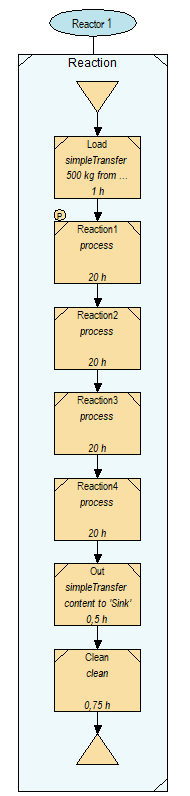In the first step of the reaction, a parameter control is used to set the duration and the resource amount for every single reaction step. Within that control it is possible to use a function to determine the resource demand. In this example, an exponential function is used to determine the flow of cooling agent through the cooling jacket. To prevent the resource from being released after every operation, the resource must be allocated permanently. In the beginning, the start value for the need of cooling agent is assigned as a permanent resource demand. After each operation the difference between the function and the previous resource amount is deallocated. The parameter control could look like this (please note that WWB.NET code is used for all code examples of this article):

``````Sub Cooling_exponential_SplitOperations(cop As OrderOperation, mop As RecipeOperation)

'f(x)=0.9^(x-25), with x in [h]

Dim n_op As Integer
Dim res As New OrderResource
Dim res_start As New OrderResource
Dim start As Double
Dim i As Integer
Dim op As OrderOperation

n_op=4 'number of operations

For i=1 To n_op
op=cop.OrderProcedure.OrderOperations("Reaction" & i)
op.Duration=t_reac(cop.Order.Material.Name, "ReactionDuration")*3600/n_op
'set the duration for every single operation
'durations are saved in parameter sheet
res=New OrderResource

If i=1 Then
start=0.9^(0-25)
'at the beginning of the first operation, the start value
'has to be assigned in this case it is for x=0
res_start.Amount=start
res_start.Allocation=0
'the resource should be allocated over the whole
'duration of the Reaction (amount>0 and allocation=0)
res_start.Resources=Resources("Cooling agent")

res.Amount=-(start-0.9^((i)*op.Duration/3600-25))
'at the end of the operation the difference between
'the start value and the first value of the function
'should be deallocated (allocation=0 and amount<0)

ElseIf i=n_op Then
'the last operation

res.Amount=-(0.9^((i-1)*op.Duration/3600-25))
'at the end of the last operation the rest of
'the resource should be deallocated
Else
res.Amount=-(start-0.9^((i)*op.Duration/3600-25))
'in every other call: difference between value
'of function for last batch(saved in CustomAttribute)
'and value for current batch is deallocated (amount<0)
End If

start=(0.9^((i)*op.Duration/3600-25))
'saving the current value of function for next loop
res.Allocation=0
res.Resources=Resources("Cooling agent")
Next

End Sub``````

The simulation result shows a decreasing amount of needed cooling agent: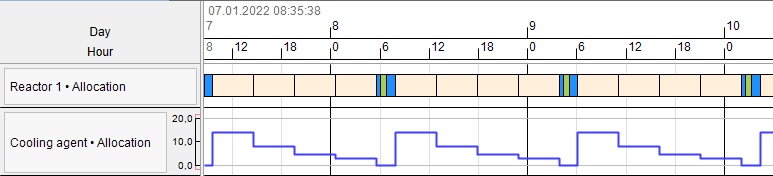This solution is the simplest possibility to use fluctuating resource demands. However, it is not very flexible, and recipes can become lengthy if a high resolution in resource demands is needed. To use a larger number of additional events, there is a second possibility:

## Second Possibility: Using a Separate Unit

The second possibility to display time-dependent resource demands is to introduce a separate unit on which additional events are created to change the resource demand over time. Let’s have a look at the example:

The cooling jacket of the reactor is modeled as a separate unit to create additional events. The trick is to use the cooling jacket in a second unit procedure which is synchronized with the start of the reaction. If you set up the recipe like this, the cooling jacket is allocated as soon as the reaction starts.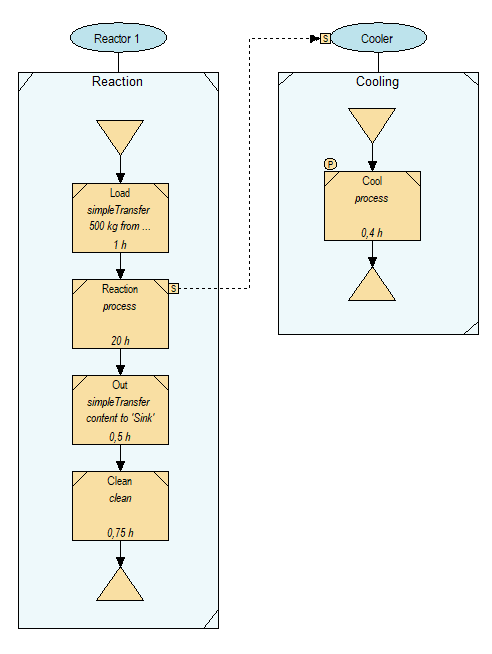With the UnitProcedure on the cooling jacket, you can now create new artificial events by setting the instances of a UnitProcedure per batch. With this, the procedure is performed several times per batch. The setting can be found in the UnitProcedure’s properties:The duration of the single operation in the cooling jacket’s UnitProcedure then needs to be adjusted according to the number of instances. In this case the duration for the operation on the cooling jacket is the reaction duration divided by 50.

The last step is to create a parameter control that assigns the resource amount to the operation. Like in the first possibility, in the beginning the start value is assigned as permanent resource demand. At the end of every operation the difference between the function and the previous resource amount is deallocated.

``````Sub Cooling_exponential(cop As OrderOperation, mop As RecipeOperation)

'f(x)=0.9^(x-25), with x in [h]

Dim batch As Integer
Dim duration As Double
Dim res As New OrderResource
Dim res_start As New OrderResource
Dim start As Double

batch=cop.OrderProcedure.Batch
'Currently executed instance of the batch
duration=cop.Duration

If batch=1 Then
start=0.9^(0-25)
'at the beginning of the first batch, the start
'value has to be assigned in this case it is at x=0
res_start.Amount=start
res_start.Allocation=0
'the resource should be allocated over the whole
'duration of the Reaction (amount>0 and allocation=0)
res_start.Resources=Resources("Cooling agent")

res.Amount=-(start-0.9^((batch)*duration/3600-25))
'at the end of the operation the difference between
'the start value and the first value of the function
'should be deallocated (allocation=0 and amount<0)

ElseIf batch=cop.ProcedureInstance.Batches Then
'the last operation
start=cop.Order.CustomAttributes("start")
res.Amount=-(0.9^((batch-1)*duration/3600-25))
'at the end of the last operation the rest
'of the resource should be deallocated

Else

start=cop.Order.CustomAttributes("start")
res.Amount=-(start-0.9^((batch)*duration/3600-25))
'in every other call: difference between value of
'function for last batch(saved in CustomAttribute)
'and value for current batch is deallocated (amount<0)

End If

cop.Order.CustomAttributes("start")=(0.9^((batch)*duration/3600-25))
'saving the current value of function for next instance
res.Allocation=0
res.Resources=Resources("Cooling agent")
End Sub``````

The result of the simulation example looks like this: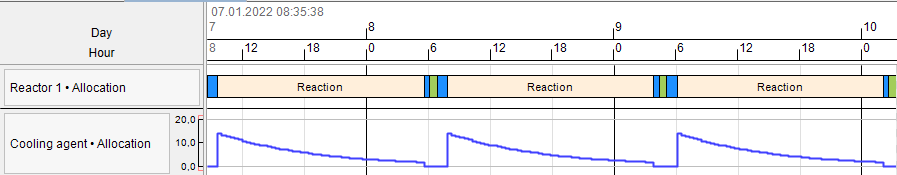In the Gantt Chart one can see that the resource is exponentially decreasing during the reaction process.

To make this even more flexible, it is also possible to assign the number of batches via VBA. In the first instance of the UnitProcedure one can set the Batches property:

``````If cop.OrderProcedure.Batch=1 Then
cop.ProcedureInstance.Batches=50
End If``````

The duration of the cooling jacket’s operation then can also be adjusted dynamically.

This method can be used if you want to detect peaks of resource demands in your simulation. To represent a limiting influence on a resource, this method is not suitable, as the resource demand is not linked to the original unit. In the case of a missing resource, only the cooling jacket would have to wait, not the Reactor itself. To make sure that the reaction operation also waits the recipe must be expanded: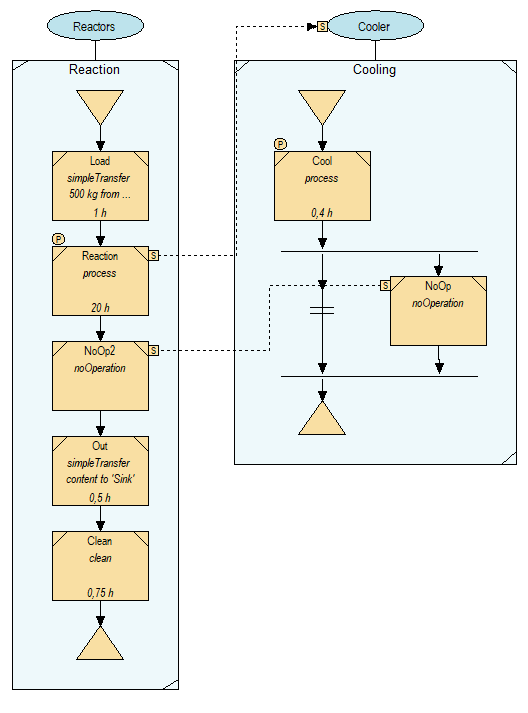One major change has been done: after the cooling, an alternative branch is introduced. The link condition checks if the current batch is the last batch of the UnitProcedure. If it is the last one, the branch with the noOperation is executed and synchronizes the operation after the reaction. By this, the next step in the recipe is delayed if the cooling has to wait for a resource.

The condition control:

``````Sub Condition_LastBatch(cond As OrderLinkCondition)
If cond.OrderOperationFrom.OrderProcedure.Batch=cond.OrderOperationFrom.ProcedureInstance.Batches Then
'if it is the last batch
cond.Value=False
Else
cond.Value=True
End If
End Sub``````

In this simulation, the amount of cooling agent is limited to 35 kg/h. After the simulation, you can see the waiting times in the Gantt chart. The waiting times on the cooling jacket are displayed at the beginning of the reaction, while the waiting times for the synchronization are displayed in the end. So, there is a little uncertainty in the depiction in the Gantt Chart, but the resource demand is displayed correctly as the resource can only be used if there is enough available. Otherwise, the operation is delayed, and the reaction is extended.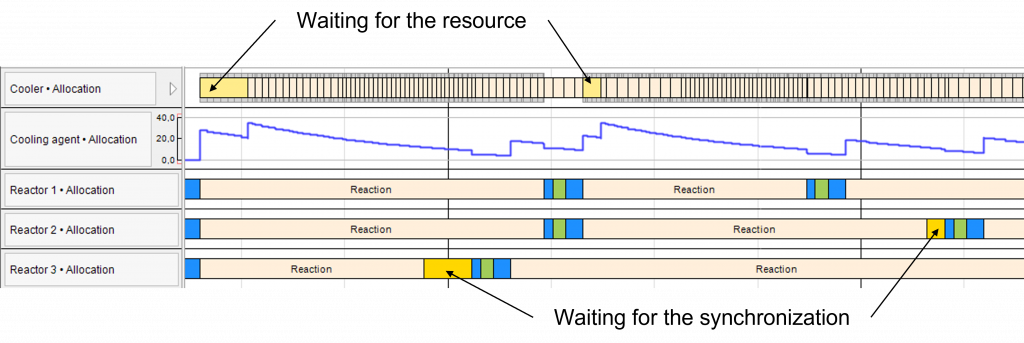## Special Case: Decreasing Resource Demands

In the described example the amount of the resource cooling agent decreases over the reaction duration. In order to model this behavior another possibility can be used. In this case no extra events have in INOSIM have to be created. The possibility to assign resources only for a part of the reaction duration (resource allocation<1) at the beginning of the operation. In this example the resource demand is changed over 50 steps according to an exponential function. The smallest value of the function that describes the needed amount of cooling agent has to be assigned for the whole duration of the reaction. The difference between the smallest value and the next bigger value of the function, will then be needed for the whole reaction duration minus the step size. In this example the difference would be added to the smallest value for 49/50 of the reaction duration. This procedure is done until the biggest value of the function, whose difference to the last value is assigned for 1/50 of the reaction duration. In VBA the behavior can be created by a loop in a parameter control:

``````Sub Cooling_exponential_ressourcesplit(cop As OrderOperation, mop As RecipeOperation)

'f(x)=0.9^(x-25) x in h

Dim duration As Double
Dim res As New OrderResource
Dim start As Double
Dim number As Integer
Dim i As Integer

number=50 'number of increments

cop.Duration=t_reac(cop.Order.Material.Name, "ReactionDuration")*3600'Duration of Reaction is saved in parameter sheet

For i=1 To number
duration=cop.Duration/number 'Duration of increment
res=New OrderResource
res.Resources=Resources("Cooling agent")

If i=1Then
res.Amount=(0.9^((number-i)*duration/3600-25)) 'first loop:smallest value that is need over the whole reaction duration (allocation:50/50=1)
Else
res.Amount=(0.9^((number-i)*duration/3600-25))-start 'difference between current function and value before, adding up to the function (allocation: 49/50; 48/50; ...)
End If

res.Allocation=1-(i-1)/number 'allocation of increment: 50/50; 49/50; 48/50; ...
start=0.9^((number-i)*duration/3600-25) 'saving value for next loop
Next

End Sub``````

In a simulation with limited amount of cooling agent, the following results can be seen in the Gantt chart: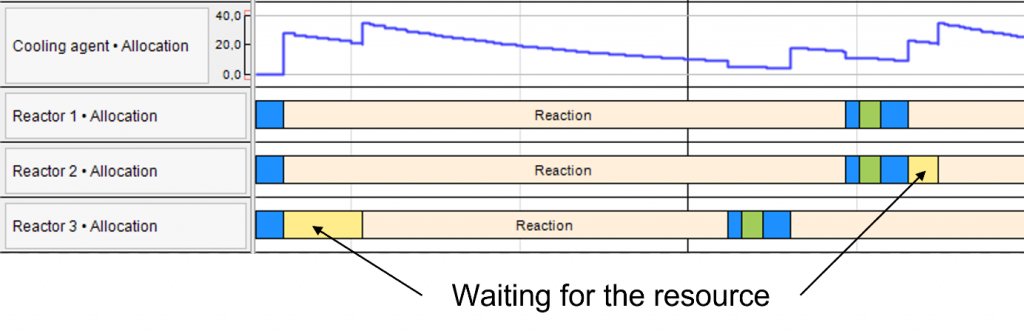This procedure can only be used for decreasing resource demands. A resource demand that is only needed for a part of an operation’s duration (allocation<1), will be used accordingly in the first part of the duration. This means that with this modeling method, a bigger resource demand can only be modeled in the beginning of an operation.

These methods for handling time dependent resource demands can be used when it is important to have a detailed resource model to identify peaks or limitations in your resources. The additional events can cause the simulation to slow down if you use a lot of them. But if there is significant need to have a detailed look, this would be a suitable workaround.

(for registered INOSIM users)

• INOSIM project
• PDF printout of this Tip & Trick

## More Questions?

Array ( [posts_per_page] => 3 [post_type] => [category__in] => Array (  => 36 ) [orderby] => rand [order] => ASC )

## More Tips & Tricks

15. March 2023

#### Preventing Runtime Errors and Custom Error Handling

This Tip & Trick describes a multitude of methods to prevent and handle runtime errors of your VBA code. You will be introduced to the…

26. November 2020

#### Fast Sorting Of Arrays

Fast Sorting Of Arrays When sorting arrays, the runtime of the applied sorting procedure may play a crucial role. For smaller amounts of data, that…

16. March 2019

#### Custom Bars

Custom Bars Custom bars are a new feature introduced in INOSIM 12. They allow you to display processes in your Gantt chart which are not…

Direct Contact